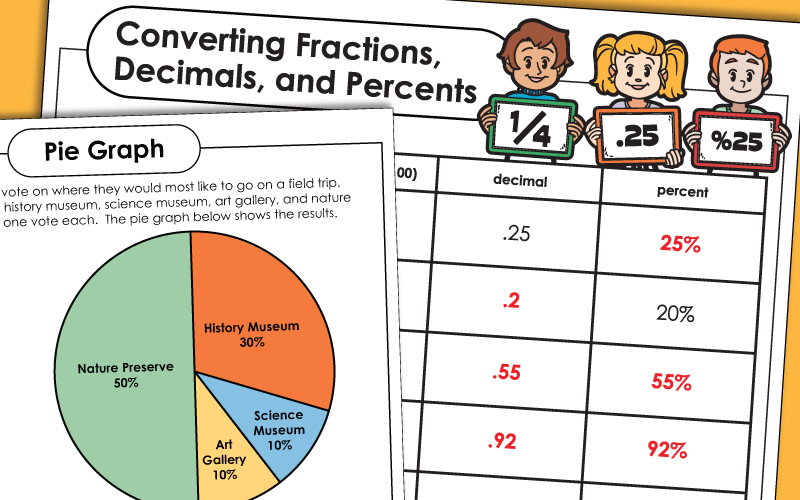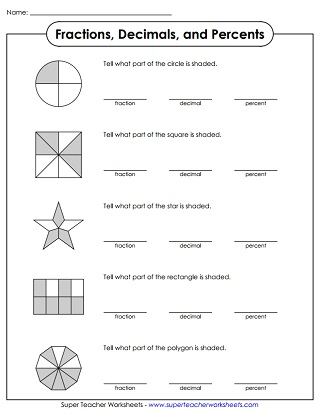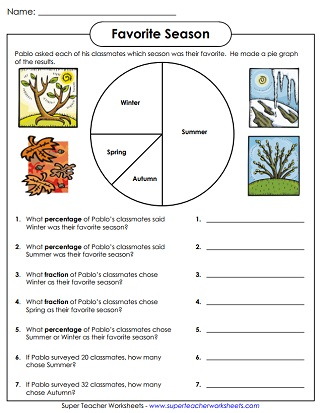# Percent Worksheets

Use these printable worksheets to teach students about percentages. Convert from fractions and decimals to percents, solve word problems, and more.## Percents (Basic)

Convert the fractions and decimals into percents and complete the table. All fractions are out of 100.
Write each percent as a fraction (and vice-versa). Includes a word problem too. All fractions are out of 100.
Tell what percentage of each picture is shaded. All pictures are divided into tenths or hundredths.
Cut out the fraction and decimal squares. Glue each next to the corresponding percent. (example: .06 = 6%)

## Percents (Intermediate)

Complete the table by writing fractions in simplest form, decimals, and percents.
Convert each fraction into a percent, then convert the percents into fractions, then solve the word problem.
Students list the percent, fraction, and decimal amounts for each of the colors in each pie graph.
Tell the percentage of each shape that is shaded. Also list the fraction and decimal amount.
Solve each percent word problem. All fractions are not out of 100, so students must have a familiarity with equivalent fractions.
In this memory matching card game, students match each percent card with the correct fraction or decimal.

Create a double number line with percentages to solve each world problem.
The number line shows the relationship between fractions and percents. Compare each percent to the fraction show. Use the symbols <, >, and =.
Use the formula to calculate your weight on other planets. example: The gravity of Venus is 90% of Earth's gravity. To calculate your weight on Venus, multiply your weight by 0.9.

## Percents: Pie Graphs

This worksheet has a pie graph titled, Favorite Seasons. Use the graph to answer the questions. Requires basic knowledge of percentages.
This circle graph shows the percentages of field trip choices. Students must interpret the graph and answer the questions. Intermediate level.
This circle chart shows how Contessa spends her money. What percentage of her money was spent on clothes and movies?
Fraction Worksheets

Try these worksheets on equivalent fractions, ordering fractions, and comparing fractions.

Decimal Worksheets

Learn about decimals to the nearest tenth and hundredth with these printables.

## Sample Worksheet ImagesMy Account
Site Information